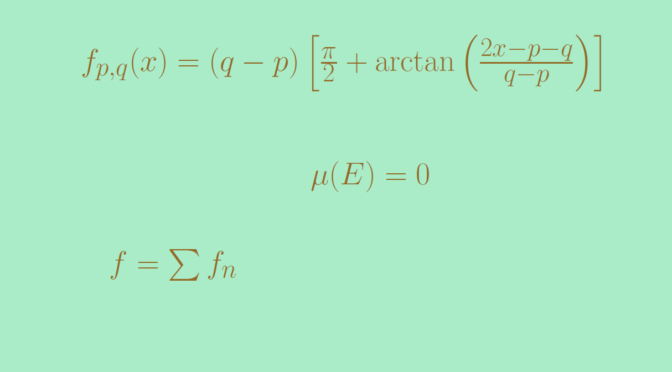# A strictly increasing continuous function that is differentiable at no point of a null set

We build in this article a strictly increasing continuous function $$f$$ that is differentiable at no point of a null set $$E$$. The null set $$E$$ can be chosen arbitrarily. In particular it can have the cardinality of the continuum like the Cantor null set.

### A set of strictly increasing continuous functions

For $$p \lt q$$ two real numbers, consider the function $f_{p,q}(x)=(q-p) \left[\frac{\pi}{2} + \arctan{\left(\frac{2x-p-q}{q-p}\right)}\right]$ $$f_{p,q}$$ is positive and its derivative is $f_{p,q}^\prime(x) = \frac{2}{1+\left(\frac{2x-p-q}{q-p}\right)^2}$ which is always strictly positive. Hence $$f_{p,q}$$ is strictly increasing. We also have $\lim\limits_{x \to -\infty} f_{p,q}(x) = 0 \text{ and } \lim\limits_{x \to \infty} f_{p,q}(x) = \pi(q-p).$ One can notice that for $$x \in (p,q)$$, $$f_{p,q}^\prime(x) \gt 1$$. Therefore for $$x, y \in (p,q)$$ distinct we have according to the mean value theorem $$\frac{f_{p,q}(y)-f_{p,q}(x)}{y-x} \ge 1$$.

### Covering $$E$$ with an appropriate set of open intervals

As $$E$$ is a null set, for each $$n \in \mathbb N$$ one can find an open set $$O_n$$ containing $$E$$ and measuring less than $$2^{-n}$$. $$O_n$$ can be written as a countable union of disjoint open intervals as any open subset of the reals. Then $$I=\bigcup_{m \in \mathbb N} O_m$$ is also a countable union of open intervals $$I_n$$ with $$n \in \mathbb N$$. The sum of the lengths of the $$I_n$$ is less than $$1$$.

$$I$$ has following property.
Let $$x \in E$$, $$U$$ be a neighbourhood of $$x$$ and let $$n \in \mathbb N$$. Then there exist $$i_1 \lt \dots \lt i_n$$ such that $$x \in I_{i_k} \subset U$$ for each $$k \in \{1, \dots,n\}$$.

Let’s prove it. As $$U$$ is supposed to be open, one can find $$m \in \mathbb N$$ large enough in order to have $$(x-2^{-m-1},x+2^{-m-1}) \subset U$$. Then $$x$$ belongs to one of the open intervals $$I_{i_1}$$ of $$O_{m+1}$$. For that $$i_1$$ we have $$I_{i_1} \subset U$$ as the length of $$I_{i_1}$$ is at most equal to $$2^{-(m+1)}$$. The prove can then be completed by induction.

### Defining the function $$f$$

Each open interval $$I_n$$ of $$I$$ can be written $$I_n=(p_n,q_n)$$ with $$p_n \lt q_n$$. For $$n \in \mathbb N$$ we denote $$f_n=f_{p_n,q_n}$$ and set $$f=\sum_{n=1}^\infty f_n$$. For all $$x \in \mathbb R$$ and $$n \in \mathbb N$$ we have $0 \le f_n(x) \le \pi (q_n-p_n) = \pi \mu(I_n),$ where $$\mu$$ denotes Lebesgue measure. By hypothesis, the series of positive numbers $$\sum \mu(I_n)$$ converges (and its sum is less than one). Therefore the series of positive functions $$\sum f_n$$ converges uniformly. Also $$f$$ is continuous and strictly increasing as the $$f_n$$ are all continuous and strictly increasing.

Let $$x \in E$$. We show that $$f$$ is not differentiable at $$x$$. According to previous paragraph, for all $$m \in \mathbb N$$ we can chose a sequence $$i_1 \lt \dots \lt i_m$$ such that $$x \in I_{i_k}$$ and $$I_{i_k} \subset (x -1/m,x+1/m)$$ for each $$k \in \{1, \dots, m\}$$. As $$I_{i_1} \cap \dots \cap I_{i_m}$$ is an open subset containing $$x$$, we can pick up $$y_m \in I_{i_1} \cap \dots \cap I_{i_m}$$ distinct of $$x$$. Then $\frac{f(y_m)-f(x)}{y_m-x} \ge \sum_{k=1}^m \frac{f_{i_k}(y_m)-f_{i_k}(x)}{y_m-x} \ge \sum_{k=1}^m 1 = m,$ according to the first paragraph. The sequence $$(y_m)$$ converges to $$x$$ and $\lim\limits_{n \to \infty} \frac{f(y_m)-f(x)}{y_m-x} = \infty.$ This proves that $$f$$ is not differentiable at $$x$$.